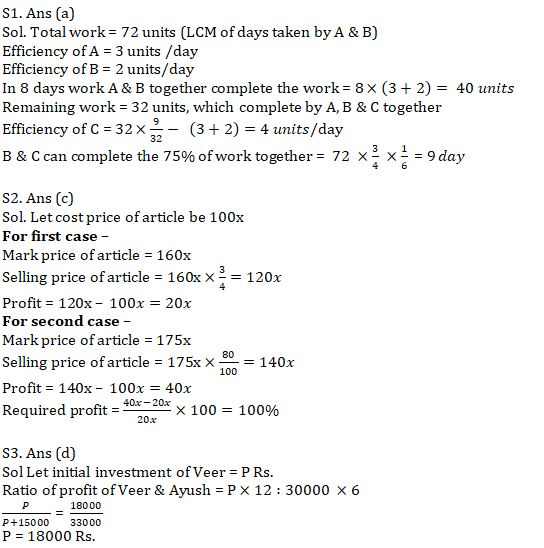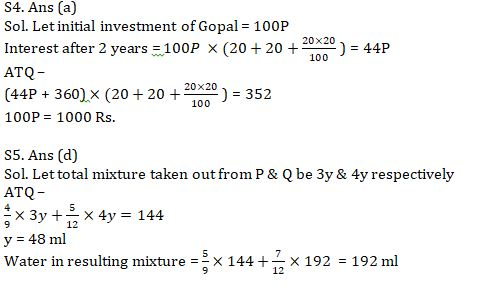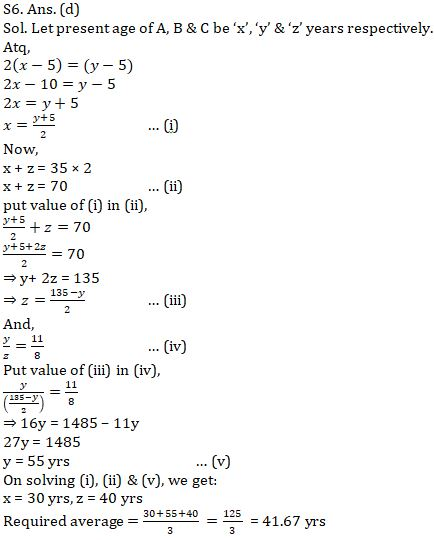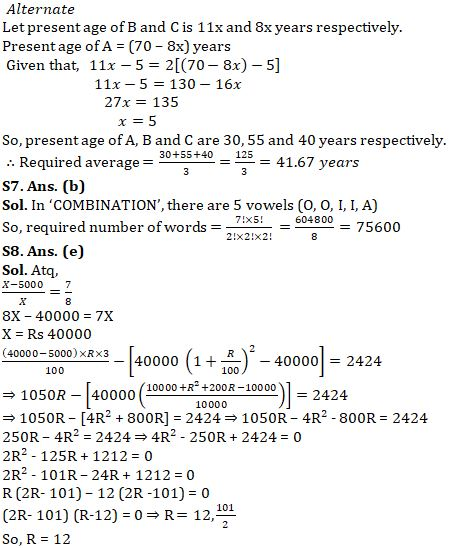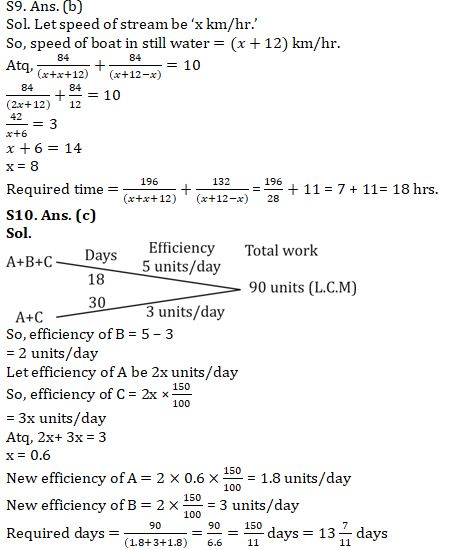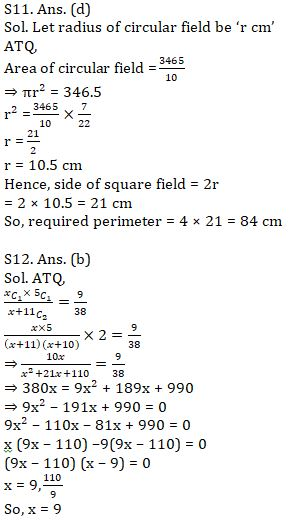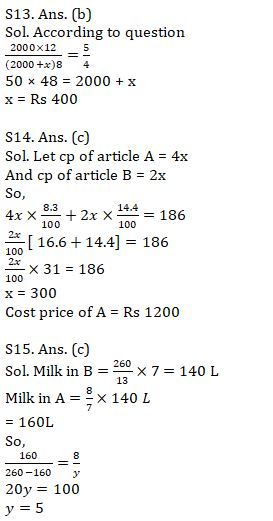Latest Banking jobs   »   Quantitative Aptitude Quiz For IBPS RRB...

# Quantitative Aptitude Quiz For IBPS RRB PO, Clerk Prelims 2021- 6th August

.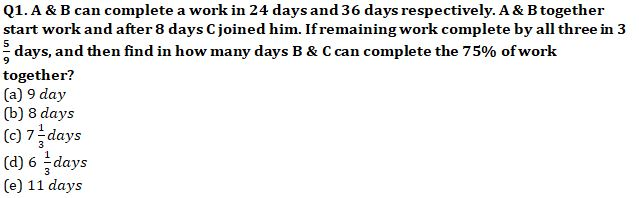Q2. A shopkeeper marks up price of an article by 60% above cost price and allow 25% discount on it. If shopkeeper marks up article by 75% above cost price and allow 20% discount on it, then find later profit is what percent more than earlier profit?
(a) 112.5%
(b) 125%
(c) 100%
(d) 75%
(e) 50%

Q3. Veer starts a business with some amount and after six months Ayush joined him with Rs. 30000. If Veer earn an annual profit of Rs. 18000 out of total profit of Rs. 33000, then find amount invested by Veer?
(a) 12000 Rs.
(b) 16000 Rs.
(c) 24000 Rs.
(d) 18000 Rs.
(e) 20000 Rs.

Q4. Gopal invested an amount in scheme which offer 20% C.I. for 2 year. He added 360 Rs. in interest earned and invested the resultant sum in same scheme for 2 more year. If he earns Rs. 352 after 2 years on this resultant sum. Then find his initial investment. (in Rs.)
(a) 1000 Rs.
(b) 1200 Rs.
(c) 800 Rs.
(d) 1600 Rs.
(e) None of these

Q5. Two mixtures P & Q of concentration 4 : 5 and 5 : 7 of juice & water poured in the ratio of 3 : 4 in a vessel. If juice in resulting mixture is 144 ml , then find quantity of water in resulting mixture?
(a) 172 ml
(b) 164 ml
(c) 200 ml
(d) 192 ml
(e) 240 ml

Q6. 5 years ago B’s age is twice of that of A and average of present age of A & C is 35 years. If ratio of present age of B to that of C is 11 : 8, then find average of present age of A, B & C.
(a) 48.67 years
(b) 34.67 years
(c) 45.33 years
(d) 41.67 years
(e) 30.33 years

Q7. Find how many words can be formed from the word ‘COMBINATION’, such that vowels will always come together.
(a) 78200
(b) 75600
(c) 64800
(d) 52600
(e) 58400

Q8. Deepak invested Rs.(X-5000) at R% p.a. SI for 3 years and Rs.X at R% p.a. CI for 2 years. If simple interest earned in 3 years is Rs.2424 more than compound interest earned in 2 years and ratio of amount invested at SI and CI is 7 : 8, then find integral value of R.
(a) 15
(b) 8
(c) 10
(d) 5
(e) 12

Q9. A boat takes a total of 10 hours to cover 84 km in upstream & 84 km in downstream. If speed of boat in still water is 12 km/hr. more than speed of stream, then find total time taken by boat to cover 196 km in downstream and 132 km in upstream.
(a) 20 hours
(b) 18 hours
(c) 22 hours
(d) 28 hours
(e) 26 hours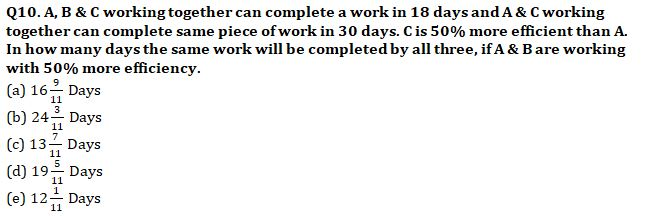Q11. Cost of paving a circular field at Rs.10/cm2 is Rs.3465. If side of square field is equal to diameter of circular field, then find perimeter of square field.
(a) 72 cm
(b) 96 cm
(c) 120 cm
(d) 84 cm
(e) 108 cm

Q12. A bag has 6 blue balls, ‘x’ red balls and 5 green balls. If two balls are picked randomly, then probability of 1 being red and 1 being green is 9/38. Find value of x.
(a) 5
(b) 9
(c) 4
(d) 7
(e) 8

Q13. A and B invested Rs 2000 and Rs (2000 +x) respectively. B withdraw from the business after 8 months. If at the end of the year profit obtained by B is 20% less than the profit obtained by A then find the value of x
(a) Rs 500
(b) Rs 400
(c) Rs 450
(d) Rs 600
(e) Rs 200

Q14. Cost price of article A is twice that of article B. If on selling A and B there is a profit of 8.3% and 14.4% respectively and total profit obtained is Rs 186, then find the cost price of article A.
(a) 1000
(b) 1100
(c) 1200
(d) 1250
(e) 1050

Q15. Two vessel A and B of equal capacity contains mixture of milk and water in the ratio 8 : y and 7 : 6 respectively. If total quality of each vessel is 260L and quantity of milk in A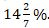. More than quantity of milk of milk in B then find y
(a) 10
(b) 4
(c) 5
(d) 6
(e) 7

Practice More Questions of Quantitative Aptitude for Competitive Exams:

###### Target IBPS RRB PO and Clerk Prelims 2021

Solutions